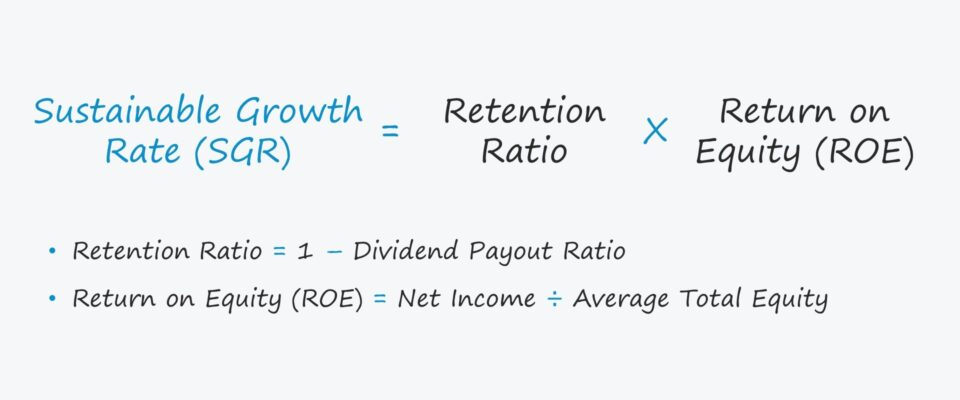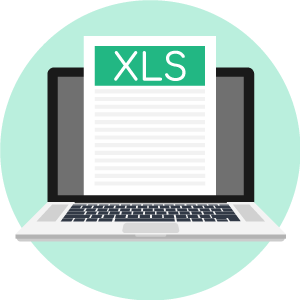# Sustainable Growth Rate (SGR)• What is the definition of the sustainable growth rate (SGR)?
• Which formula calculates the sustainable growth rate (SGR)?
• What does the sustainable growth rate (SGR) measure?
• How is the sustainable growth rate different from the internal growth rate?

## Sustainable Growth Rate (SGR) Formula

The sustainable growth rate represents the rate at which a company can maintain its growth without requiring additional financing from external sources.

The sustainable growth rate is a company’s growth rate that can continue under its current capital structure.

The capital structure refers to how a company is funding its current growth (and future growth), i.e. the mixture of debt and equity to fund operations and asset purchases.

Most early-stage companies that are either unprofitable or barely profitable are self-funded until reaching the point where external financing becomes an absolute necessity, typically in the form of equity issuances.

Mature companies that are profitable and have more established market positions can opt to fund themselves from three sources:

1. Internal Funding:: Companies can use their retained earnings (i.e. the accumulated net earnings not paid out as dividends to shareholders).
2. Equity Issuances: Companies can raise capital by selling off pieces of ownership to institutional and/or retail investors for capital.
3. Debt Issuances: Companies can raise capital via borrowing agreements, where lenders provide capital in exchange for interest payments and the return of principal at maturity.
###### Sustainable Growth Rate & Company Lifecycle

The sustainable growth rate (SGR) can be a useful indicator of which stage of its life cycle a company is currently in. In general, the higher the sustainable growth rate (SGR), the greater its potential upside.

But greater potential returns cannot come without more downside risks, e.g. earnings volatility and default risk. If the sustainable growth rate (SGR) is adequate to management and investors, there is likely no reason to take on further leverage.

Once companies approach the later stages in their life cycle, maintaining a high SGR over the long run can be challenging as the opportunities for expansion and growth eventually fade with time.

Plus, consumer demands continuously change, and new entrants will inevitably attempt to disrupt the market to steal market share from existing incumbents, resulting in higher capital expenditures (CapEx) and research & development (R&D).

## Sustainable Growth Rate (SGR) Formula

The formula for calculating the sustainable growth rate (IGR) consists of three steps:

### Sustainable Growth Rate Formula

The formula to calculate the sustainable growth rate (SGR) is shown below.

• Sustainable Growth Rate (SGR) = Retention Rate × Return on Equity

Where:

• Retention Rate = (1 – Dividend Payout Ratio)
• Return on Equity = Net Income ÷ Average Shareholders’ Equity

The dividend payout ratio is the percentage of earnings per share (EPS) paid to shareholders as dividends – thus, if we subtract the percentage paid out as dividends from one, we are left with the retention ratio.

The retention ratio is the portion of net income that is retained as opposed to being paid out as dividends to compensate shareholders.

The return on equity (ROE) measures a company’s profitability based on each dollar of equity investment contributed by its shareholder base.

For example, if a company has a return on equity (ROE) of 10% and a dividend payout ratio of 20%, the sustainable growth rate is 8%.

• Sustainable Growth Rate (SGR) = (1 – 20%) × 10%
• SGR = 0.80 x 0.10 = 8%

Here, the company can grow at 8% per year if the capital structure is left unadjusted by management and operations remain consistent with historical performance.

## Sustainable Growth Rate vs Internal Growth Rate

The internal growth rate is the maximum rate at which a company can grow without relying on external financing sources (e.g. equity or debt issuances).

The IGR assumes that operations will be entirely self-funded by the company’s retained earnings.

In contrast, the sustainable growth rate (SGR) includes the impact of external financing, but the existing capital structure is kept constant.

Since the sustainable growth rate considers the use of leverage – which increases the potential upside in returns and the potential losses – the SGR should be higher than the IGR.

## Sustainable Growth Rate (SGR) Calculator – Excel Template

We’ll now move to a modeling exercise, which you can access by filling out the form below.Submitting ...

## Sustainable Growth Rate (SGR) Example Calculation

Suppose a company has the following financials.

• Net Income to Common Shareholders = \$50 million
• Weighted Average Shares Outstanding = 10 million
• Annual Dividend = \$25 million

The earnings per share (EPS) and dividend per share (DPS) can be calculated using those assumptions.

• Earnings Per Share (EPS) = \$50 million ÷ 10 million = \$5.00
• Dividend Per Share (DPS) = \$25 million ÷ 10 million = \$2.50

Side Note: The reason we’re using “Net Income to Common Shareholders” rather than just “Net Income” is that the net income attributable to preferred stockholders should not be included (e.g. preferred dividends).

Next, the retention ratio can be calculated by subtracting the payout ratio from one:

• Retention Ratio = 1 – (\$2.50 ÷ \$5.00) = 50%

Considering that high payout ratios are often signs of a highly profitable company with a stable outlook, it is safe to assume that our company is relatively mature.

Moving on, we’ll calculate the return on equity (ROE) next by dividing net income by the average shareholder’s equity, which we’ll assume to be \$200 million.

• Return on Equity (ROE) = \$50 million ÷ \$200 million
• ROE = 25%

Finally, the sustainable growth rate (SGR) can be calculated by multiplying the retention ratio by the ROE.

• Sustainable Growth Rate (SGR) = 50% × 25%
• SGR = 12.5%Inline FeedbacksLearn Financial Modeling Online

Everything you need to master financial and valuation modeling: 3-Statement Modeling, DCF, Comps, M&A and LBO.

X

The Wall Street Prep Quicklesson Series

7 Free Financial Modeling Lessons

Get instant access to video lessons taught by experienced investment bankers. Learn financial statement modeling, DCF, M&A, LBO, Comps and Excel shortcuts.